Next: 4.7 Straylight Up: 4. Instrumental Characteristics Previous: 4.5 Optical Performance

Subsections

# 4.6 Spectral Performance

## 4.6.1 PHT-S instrumental line profile

The PHT-S instrumental line profiles have been measured in laboratory. The profiles exhibit a Gaussian shape to good approximation. For the PHT-SS pixels the FWHM is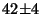nm, for SL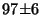nm. The physical distance between adjacent pixels is 350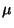m which corresponds to 38.3 nm and 91.8 nm in wavelength for SS and SL respectively, assuming a linear scale. The physical size of a pixel in dispersion direction is 310m which corresponds to a width in wavelength of 33.9 and 81.3 nm for SS and SL. In Table 4.5 we have summarized the laboratory data. Also listed are the fraction of the incident power measured by the detector pixel in the case the line is centred on a pixel and in case the line falls exactly in between two pixels of the PHT-S arrays. For these values it is assumed that the detectors have an ideal flat-topped responsivity profile in dispersion direction. Since this is not the case (see Section 4.5.1.3) the ratio between the centre and adjacent pixel in case the line is centred on the pixel is somewhat higher. For the values in Table 4.5 an accurate approximation for the wavelength scale is used. For the exact scale see Section 4.6.2. Under the given assumptions, a 10% variation in the FWHM changes the fraction in the centre pixel by less than 7%.

 parameter SS SL unit description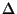x 350 350m physical distance between pixels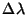38.3 91.8 nm wavelength difference corresponding to pixel distancel 310 310m physical pixel size in Z-direction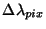33.9 81.3 nm pixel width in wavelength FWHM 42 97 nm mean full width at half power of profile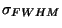4 6 nm uncertainty in FWHM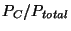0.73 0.74 - power fraction measured on pixel if line is centred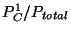0.09 0.08 - power fraction measured on adjacent pixel if line is centred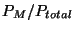0.43 0.43 - power fraction on pixel if line is centred between two pixels

## 4.6.2 PHT-S wavelength calibration

The PHT-S wavelength scale, i.e. the relation between pixel number and wavelength, is approximately linear. In orbit calibration confirms the presence of a small second order term. The wavelength scale has been calibrated to an accuracy of less than 1/10 of a pixel (see also Table 4.5) from the analysis of several targets with well defined emission lines. A polynomial fit to the wavelength scale for PHT-SS and PHT-SL gives: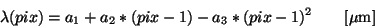(4.7)

where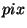is the integer pixel number in the SS or SL array, counting from 1 to 64 for both SS and SL. The coefficients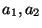, and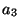are presented in Table 4.6.

 Coefficient SS SL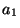2.4687 5.8396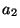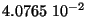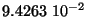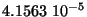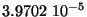Next: 4.7 Straylight Up: 4. Instrumental Characteristics Previous: 4.5 Optical Performance
ISO Handbook Volume IV (PHT), Version 2.0.1, SAI/1999-069/Dc